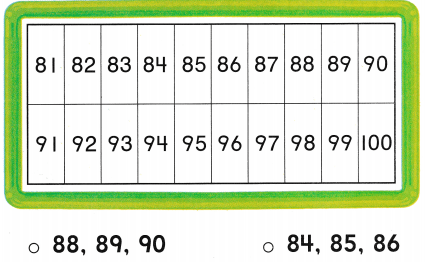# Texas Go Math Kindergarten Lesson 16.2 Answer Key Count to 100 by Ones

Refer to our Texas Go Math Kindergarten Answer Key Pdf to score good marks in the exams. Test yourself by practicing the problems from Texas Go Math Kindergarten Lesson 16.2 Answer Key Count to 100 by Ones.

## Texas Go Math Kindergarten Lesson 16.2 Answer Key Count to 100 by Ones

Essential Question
How does the order of numbers help you count to 100 by ones?

Explore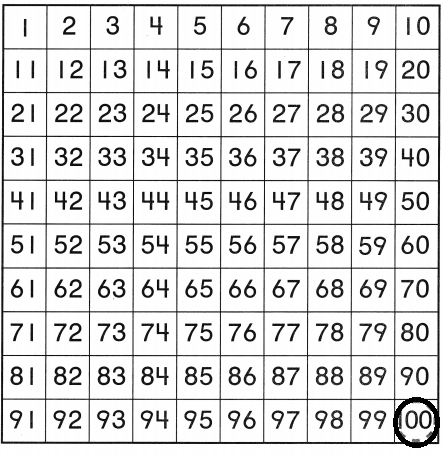Explanation:
Circled the number 100
started counting from 1 and ended at 100

Directions
Point to each number as you count to 100. Trace the circle around the number 100.

Share and Show

Question 1.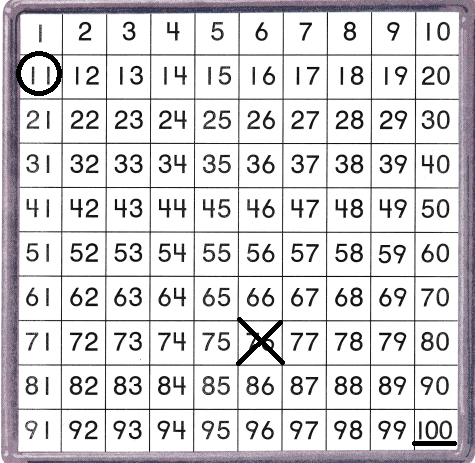Explanation:
Underlined the number 100
circled the number 11 and marked X on number 76

Directions
1. Point to each number as you count to 100, Draw a line under the number 100. Circle the number II. Begin with II and count forward to 100. Mark an X on the number 76. Count forward again from 76 to 100.

Question 2.Explanation:
Randomly selected the number 23
The number after 70 is 71
crossed the number 49 and underlined the number 100.

Directions
2. Point to each number as you count to 100. Look away and point to any number. Circle that number. Count forward to 100 from that number. Draw a line under the number 100. Mark on X on the number that comes right after 49. Count forward again from that number to 100. Find the number that comes right after 70. Color the box with that number red. Count forward from that number to 100.

Home Activity

• Show your child a calendar, Point to a number on the calendar Have your child count forward from that number to the last number of the month.

Problem Solving

Question 3.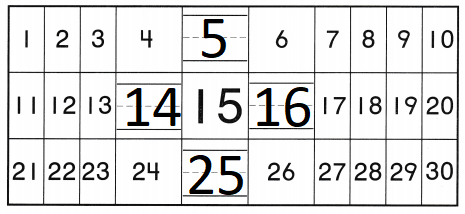Explanation:
The number after 4 is 5
14 after 15 and then 16
The number after 24 is 25.

Question 4.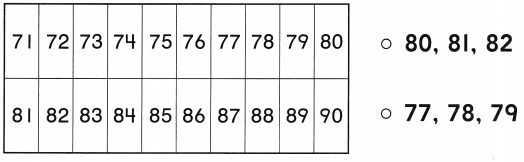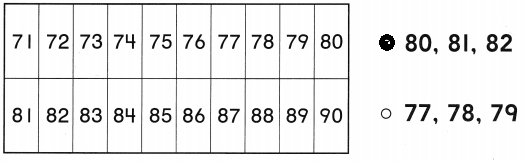Explanation:
the number after 79 are 80 , 81 , 82
so marked the numbers.

Directions
3. Place your finger on the number 15. Write to show the numbers that are neighbors to the number 15. Compare each number to 15 using greater than or less than. 4. Choose the correct answer. Point to each number as you count. Which three numbers come after 79?

### Texas Go Math Kindergarten Lesson 16.2 Homework and Practice

Question 1.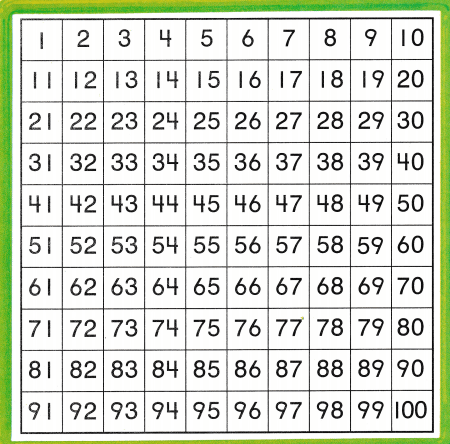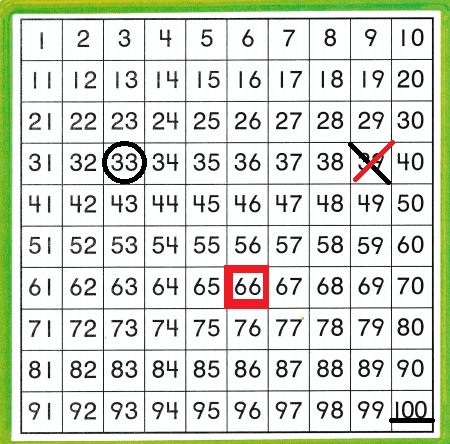Explanation:
Randomly circled the number 33
underlined the number 100
The number after 65 is 66
Crossed the number 39

Directions
1. Point to each number as you count to 100. Look away and point to any number. Circle that number. Count forward to 100 from that number. Draw a line under the number 100, Mark on X on the number that comes right after 39, Count forward from that number to 100. Find the number that comes right after 65. Color the box with that number red. Count forward from that number to 100.

Lesson Check

Question 2.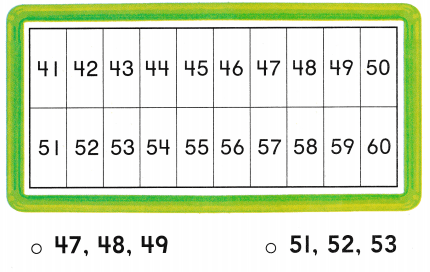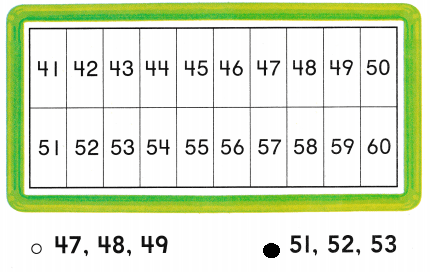Explanation:
The three numbers after 50 are 51 , 52 , 53
so, marked those 3 numbers.

Question 3.